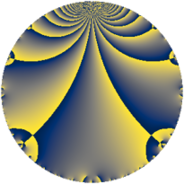# Properties

 Label 2800.2.a.gLevel $2800$ Weight $2$ Character orbit 2800.a Self dual yes Analytic conductor $22.358$ Analytic rank $1$ Dimension $1$ CM no Inner twists $1$

# Related objects

## Newspace parameters

 Level: $$N$$ $$=$$ $$2800 = 2^{4} \cdot 5^{2} \cdot 7$$ Weight: $$k$$ $$=$$ $$2$$ Character orbit: $$[\chi]$$ $$=$$ 2800.a (trivial)

## Newform invariants

 Self dual: yes Analytic conductor: $$22.3581125660$$ Analytic rank: $$1$$ Dimension: $$1$$ Coefficient field: $$\mathbb{Q}$$ Coefficient ring: $$\mathbb{Z}$$ Coefficient ring index: $$1$$ Twist minimal: no (minimal twist has level 14) Fricke sign: $$1$$ Sato-Tate group: $\mathrm{SU}(2)$

## $q$-expansion

 $$f(q)$$ $$=$$ $$q - 2q^{3} + q^{7} + q^{9} + O(q^{10})$$ $$q - 2q^{3} + q^{7} + q^{9} + 4q^{13} - 6q^{17} - 2q^{19} - 2q^{21} + 4q^{27} - 6q^{29} + 4q^{31} - 2q^{37} - 8q^{39} + 6q^{41} + 8q^{43} - 12q^{47} + q^{49} + 12q^{51} - 6q^{53} + 4q^{57} + 6q^{59} + 8q^{61} + q^{63} - 4q^{67} - 2q^{73} - 8q^{79} - 11q^{81} - 6q^{83} + 12q^{87} - 6q^{89} + 4q^{91} - 8q^{93} + 10q^{97} + O(q^{100})$$

## Embeddings

For each embedding $$\iota_m$$ of the coefficient field, the values $$\iota_m(a_n)$$ are shown below.

For more information on an embedded modular form you can click on its label.

Label $$\iota_m(\nu)$$ $$a_{2}$$ $$a_{3}$$ $$a_{4}$$ $$a_{5}$$ $$a_{6}$$ $$a_{7}$$ $$a_{8}$$ $$a_{9}$$ $$a_{10}$$
1.1
 0
0 −2.00000 0 0 0 1.00000 0 1.00000 0
 $$n$$: e.g. 2-40 or 990-1000 Significant digits: Format: Complex embeddings Normalized embeddings Satake parameters Satake angles

## Atkin-Lehner signs

$$p$$ Sign
$$2$$ $$-1$$
$$5$$ $$1$$
$$7$$ $$-1$$

## Inner twists

This newform does not admit any (nontrivial) inner twists.

## Twists

By twisting character orbit
Char Parity Ord Mult Type Twist Min Dim
1.a even 1 1 trivial 2800.2.a.g 1
4.b odd 2 1 350.2.a.f 1
5.b even 2 1 112.2.a.c 1
5.c odd 4 2 2800.2.g.h 2
12.b even 2 1 3150.2.a.i 1
15.d odd 2 1 1008.2.a.h 1
20.d odd 2 1 14.2.a.a 1
20.e even 4 2 350.2.c.d 2
28.d even 2 1 2450.2.a.t 1
35.c odd 2 1 784.2.a.b 1
35.i odd 6 2 784.2.i.i 2
35.j even 6 2 784.2.i.c 2
40.e odd 2 1 448.2.a.g 1
40.f even 2 1 448.2.a.a 1
60.h even 2 1 126.2.a.b 1
60.l odd 4 2 3150.2.g.j 2
80.k odd 4 2 1792.2.b.c 2
80.q even 4 2 1792.2.b.g 2
105.g even 2 1 7056.2.a.bd 1
120.i odd 2 1 4032.2.a.r 1
120.m even 2 1 4032.2.a.w 1
140.c even 2 1 98.2.a.a 1
140.j odd 4 2 2450.2.c.c 2
140.p odd 6 2 98.2.c.b 2
140.s even 6 2 98.2.c.a 2
180.n even 6 2 1134.2.f.f 2
180.p odd 6 2 1134.2.f.l 2
220.g even 2 1 1694.2.a.e 1
260.g odd 2 1 2366.2.a.j 1
260.u even 4 2 2366.2.d.b 2
280.c odd 2 1 3136.2.a.z 1
280.n even 2 1 3136.2.a.e 1
340.d odd 2 1 4046.2.a.f 1
380.d even 2 1 5054.2.a.c 1
420.o odd 2 1 882.2.a.i 1
420.ba even 6 2 882.2.g.c 2
420.be odd 6 2 882.2.g.d 2
460.g even 2 1 7406.2.a.a 1

By twisted newform orbit
Twist Min Dim Char Parity Ord Mult Type
14.2.a.a 1 20.d odd 2 1
98.2.a.a 1 140.c even 2 1
98.2.c.a 2 140.s even 6 2
98.2.c.b 2 140.p odd 6 2
112.2.a.c 1 5.b even 2 1
126.2.a.b 1 60.h even 2 1
350.2.a.f 1 4.b odd 2 1
350.2.c.d 2 20.e even 4 2
448.2.a.a 1 40.f even 2 1
448.2.a.g 1 40.e odd 2 1
784.2.a.b 1 35.c odd 2 1
784.2.i.c 2 35.j even 6 2
784.2.i.i 2 35.i odd 6 2
882.2.a.i 1 420.o odd 2 1
882.2.g.c 2 420.ba even 6 2
882.2.g.d 2 420.be odd 6 2
1008.2.a.h 1 15.d odd 2 1
1134.2.f.f 2 180.n even 6 2
1134.2.f.l 2 180.p odd 6 2
1694.2.a.e 1 220.g even 2 1
1792.2.b.c 2 80.k odd 4 2
1792.2.b.g 2 80.q even 4 2
2366.2.a.j 1 260.g odd 2 1
2366.2.d.b 2 260.u even 4 2
2450.2.a.t 1 28.d even 2 1
2450.2.c.c 2 140.j odd 4 2
2800.2.a.g 1 1.a even 1 1 trivial
2800.2.g.h 2 5.c odd 4 2
3136.2.a.e 1 280.n even 2 1
3136.2.a.z 1 280.c odd 2 1
3150.2.a.i 1 12.b even 2 1
3150.2.g.j 2 60.l odd 4 2
4032.2.a.r 1 120.i odd 2 1
4032.2.a.w 1 120.m even 2 1
4046.2.a.f 1 340.d odd 2 1
5054.2.a.c 1 380.d even 2 1
7056.2.a.bd 1 105.g even 2 1
7406.2.a.a 1 460.g even 2 1

## Hecke kernels

This newform subspace can be constructed as the intersection of the kernels of the following linear operators acting on $$S_{2}^{\mathrm{new}}(\Gamma_0(2800))$$:

 $$T_{3} + 2$$ $$T_{11}$$ $$T_{13} - 4$$

## Hecke characteristic polynomials

$p$ $F_p(T)$
$2$ $$T$$
$3$ $$2 + T$$
$5$ $$T$$
$7$ $$-1 + T$$
$11$ $$T$$
$13$ $$-4 + T$$
$17$ $$6 + T$$
$19$ $$2 + T$$
$23$ $$T$$
$29$ $$6 + T$$
$31$ $$-4 + T$$
$37$ $$2 + T$$
$41$ $$-6 + T$$
$43$ $$-8 + T$$
$47$ $$12 + T$$
$53$ $$6 + T$$
$59$ $$-6 + T$$
$61$ $$-8 + T$$
$67$ $$4 + T$$
$71$ $$T$$
$73$ $$2 + T$$
$79$ $$8 + T$$
$83$ $$6 + T$$
$89$ $$6 + T$$
$97$ $$-10 + T$$